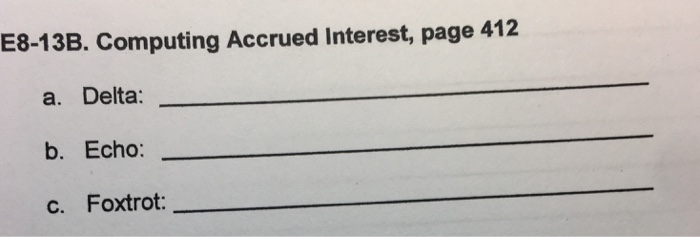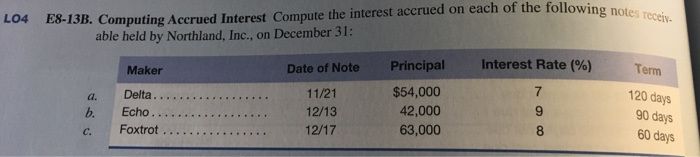# Question & Answer: E8-13B. Computing Accrued Interest, page 412 a. Delta: b. Echo c. Foxtrot:…..E8-13B. Computing Accrued Interest, page 412 a. Delta: b. Echo c. Foxtrot:

the following table shows the computation of accrued interest on notes receivable:

Don't use plagiarized sources. Get Your Custom Essay on
Question & Answer: E8-13B. Computing Accrued Interest, page 412 a. Delta: b. Echo c. Foxtrot:…..
GET AN ESSAY WRITTEN FOR YOU FROM AS LOW AS \$13/PAGE

interest amount = note amount * interest rate * (number of days till 31st december)/ number of days in the year

(let us assume the year has 360 days).

 a.Delta number of days = 10 days in november + 31 days in december =>41 days Interest = \$54,000*7%*41/360 =>\$430.5 b.Echo number of days = 19 days in december \$42,000*9%*19/360=>\$199.50 C.Foxtrot number of days = 15 days in december \$63,000*8%*15/360=>\$210